# Segal-Shale-Weil representation

A representation of groups arising in both number theory and in physics. For number theorists, the seminal paper is that of A. Weil, [a1]. He cites earlier papers of I. Segal and D. Shale as precedents, and the deep work of C.L. Siegel on theta-series as inspiration.

Letbe a group with centresuch thatis Abelian, and letbe a unitary character of(cf. also Character of a group). If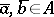, choose representativesand note thatis independent of the choice of representatives. This is a skew-symmetric bilinear pairing. One assumes that this pairing is non-degenerate. The Stone–von Neumann theorem asserts thathas a unique irreducible representationwith central character. Furthermore, the representation may be constructed as follows. Letbe a Lagrangian subgroup, that is, any subgroup ofcontainingsuch that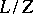is a maximal subgroup ofon which the form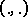is trivial. Extendtoin an arbitrary manner, then induce. This gives a model for.

Letbe a group of automorphisms ofwhich acts trivially on(cf. also Automorphism). If, the Stone–von Neumann theorem implies that. Let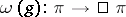be an intertwining mapping, well defined up to constant multiple (cf. also Intertwining operator). Thenis a projective representation of.

For example, letbe a local field and letbe a vector space overendowed with a non-degenerate skew-symmetric bilinear form. Its dimension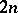is even, and the automorphism group of the form is the symplectic group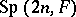. One can construct a "Heisenberg group"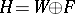with the multiplication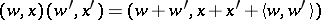. Choosing any non-trivial additive character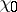of, let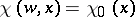. Then the hypotheses of the Stone–von Neumann theorem are satisfied. As the Lagrangian subgroup ofone may take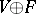, whereis any maximal isotropic subspace of. Then the induced model ofdescribed above may be realized as the Schwartz space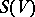. The Segal–Shale–Weil representation is the resulting projective representation of. It may be interpreted as a genuine representation of a covering group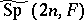, the so-called metaplectic group.

Now letbe a global field,its adèle ring (cf. also Adèle), and letandbe as before. Then one may construct a similar representationofon the Schwartz space. If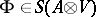, let. This linear form is invariant under the action of, generalizing the Poisson summation formula. This implies that the representationis automorphic. The corresponding automorphic forms are theta-functions (cf. Theta-function), having their historical origins in the work of C.G.J. Jacobi and Siegel. As Weil observed, the automorphicity of this representation is closely related to the quadratic reciprocity law.

Later authors, notably R. Howe [a2], have emphasized the theory of dual reductive pairs. When a pair of reductive groups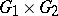embeds in, each being the centralizer of the other (cf. also Centralizer), thensets up a correspondence between representations of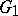and representations of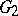. This works at the level of automorphic forms and gives instances of Langlands functoriality, including some historically important ones such as quadratic base change (cf. also Base change). See [a3]. The use of the Weil representation in [a4] to construct automorphic forms and representations may be understood as arising from the dual reductive pairsand. The dual pair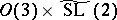underlies the important work of J.-L. Waldspurger [a5] on automorphic forms of half-integral weight.

In recent years (as of 2000) it has been noted that since the Segal–Shale–Weil representation is the minimal representation of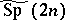, that is, the representation with smallest Gel'fand–Kirillov dimension, minimal representations of other groups can play a similar role. Many interesting examples may be found in the exceptional groups (cf. also Lie algebra, exceptional). The possibly first paper where this phenomenon was noted was [a6]. Many interesting examples come from the exceptional groups. There is much current literature on this subject, but for typical papers see [a7] and [a8]. Dual pairs in the exceptional groups were classified in [a9].

For further references see [a10].